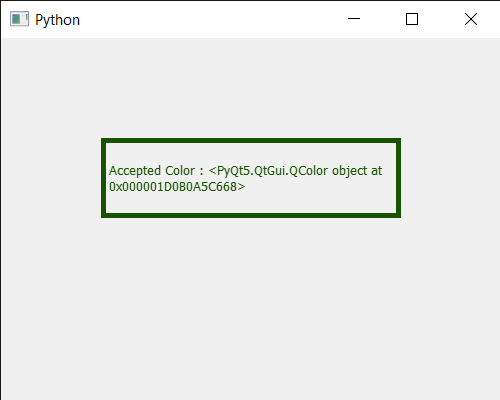Related Articles
PyQt5 QColorDialog – Accepted Signal
• Last Updated : 01 Aug, 2020

In this article, we will see how we can get the accepted signal of the QColorDialog widget. This signal is emitted when the dialog has been accepted either by the user or by calling `accept()` or `done()` with the QDialog::Accepted argument.

Note : This signal is not emitted when hiding the dialog with hide() or setVisible(false). This includes deleting the dialog while it is visible.

In order to do this we use `accepted` method with the QColorDialog object

Syntax : dialog.accepted.connect(lambda: print(“Accepted Signal”)

Argument : It takes method as argument

Action Performed : It will call the passed method when accepted signal emitted

Below is the implementation

 `# importing libraries``from` `PyQt5.QtWidgets ``import` `*``from` `PyQt5 ``import` `QtCore, QtGui``from` `PyQt5.QtGui ``import` `*``from` `PyQt5.QtCore ``import` `*``import` `sys`` ` ` ` `class` `Window(QMainWindow):`` ` `    ``def` `__init__(``self``):``        ``super``().__init__()`` ` `        ``# setting title``        ``self``.setWindowTitle(``"Python "``)`` ` `        ``# setting geometry``        ``self``.setGeometry(``100``, ``100``, ``500``, ``400``)`` ` `        ``# calling method``        ``self``.UiComponents()`` ` `        ``# showing all the widgets``        ``self``.show()`` ` ` ` `    ``# method for components``    ``def` `UiComponents(``self``):`` ` `        ``# creating a QColorDialog object``        ``dialog ``=` `QColorDialog(``self``)`` ` `        ``# setting current color to the dialog``        ``dialog.setCurrentColor(Qt.red)`` ` ` ` `        ``dialog.accepted.connect(``lambda``: ``print``(``"Accpeted Signal"``))`` ` `        ``# making color done``        ``# accepting the color``        ``dialog.done(``1``)`` ` `        ``# executing the dialog``        ``dialog.exec_()`` ` ` ` `        ``# creating label``        ``label ``=` `QLabel(``"GeeksforGeeks"``, ``self``)`` ` `        ``# setting geometry to the label``        ``label.setGeometry(``100``, ``100``, ``300``, ``80``)`` ` `        ``# making label multi line``        ``label.setWordWrap(``True``)`` ` `        ``# setting stylesheet of the label``        ``label.setStyleSheet(``"QLabel"``                            ``"{"``                            ``"border : 5px solid black;"``                            ``"}"``)`` ` `        ``# getting the selected color``        ``color ``=` `dialog.selectedColor()`` ` `        ``# setting graphic effect to the label``        ``graphic ``=` `QGraphicsColorizeEffect(``self``)`` ` `        ``# setting color to the graphic``        ``graphic.setColor(color)`` ` `        ``# setting graphic to the label``        ``label.setGraphicsEffect(graphic)`` ` `        ``# setting text to the label``        ``label.setText(``"Accepted Color : "` `+` `str``(color))`` ` ` ` ` ` `# create pyqt5 app``App ``=` `QApplication(sys.argv)`` ` `# create the instance of our Window``window ``=` `Window()`` ` `# start the app``sys.exit(App.``exec``())`

Output :

```Accpeted Signal
Accpeted Signal```Attention geek! Strengthen your foundations with the Python Programming Foundation Course and learn the basics.

To begin with, your interview preparations Enhance your Data Structures concepts with the Python DS Course. And to begin with your Machine Learning Journey, join the Machine Learning – Basic Level Course

My Personal Notes arrow_drop_up